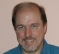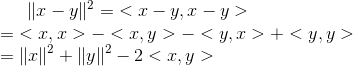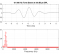## Similarity between two data pointsStarted by 7 years ago8 replieslatest reply 7 years ago1071 views

Hello All,

What is the best way to find of if two data points are similar?

For example, I have a point X with 3 features, point Y with similar 3 features and a point Z. One way is to measure the Euclidean Distance and two points with smallest distance are similar.

But can I use correlation between X, Y and Z to find out if they are similar?

Best Regards

Sia

[ - ]Hello Sia:

Other posts are correct. There are also other considerations such as whether the vector you are observing has been properly normalized so that you are comparing apples to apples.

You didn't define "how similar is similar" ... whether similarity is spherical, rectangular, cubic, etc. A very vague problem statement.

You also didn't state whether "similar" is "in reference to" a "standard" vector, or relative to each other. Similarity at a "distance" of one billion miles is a lot different than similarity at a "distance" of one millimeter ... so you also didn't state the magnitudes of your vectors.

For digital demodulators in radio receiving equipment, similar is defined as a percentage versus a reference vector, the reference vector being the "perfect" vector.

Good luck ...

[ - ]Hi Sia,

Note that for points normalized by their norm, minimizing Euclidean distance is is equivalent to maximizing cross-correlation (aka "vector cosine"):

\|x-y\|^2 = \; <x-y,x-y> \\

= \; <x,x> - <x,y> - <y,x> + <y,y> \\

= \|x\|^2 + \|y\|^2 - 2<x,y>(image courtesy of https://www.codecogs.com/latex/eqneditor.php)

- Julius

[ - ]Thanks JOS -- I intuitively knew that there was a strong relationship between correlation and Euclidean distance, but I didn't want to say anything at risk of putting my foot in my mouth, or take the time to go look it up.

It's nice when someone else can do my cross-referencing for me!

[ - ]Hi, Sia:

What is the data type (i.e. real-value, categorical, etc.) of each of the three features for each point?

Michael.

[ - ]The data type is real-value.

[ - ]First, I suspect that with just three dimensions, "correlation" between X, Y and Z will boil down to either the Elucidean distance, or to some other simpler measure that depends on how you define "correlation".

Second, what "similar" means for your problem really depends on what your problem is.  A problem in data transmission & reception may say that the minimum of ||X - a*Z|| over all real a is "most similar", where a problem in locating a point in space would say that the Euclidean distance is, indeed, the measure of similarity.

Sorry for the vague answer, but I really think that you need to define the term "similar" in the context of your problem before you can get a sensible answer.

[ - ]I understand your point from communication perspective. But the term "similar" is not defined in the question. So I have to assume or define it. I can solve it using Euclidean distance.

But I wanted to know if correlation can be applied. For example, cross-correlation is used in communication to get channel impulse response.

[ - ]